Courses

# Test: Temperature

## 10 Questions MCQ Test Topicwise Question Bank for Mechanical Engineering | Test: Temperature

Description
This mock test of Test: Temperature for Mechanical Engineering helps you for every Mechanical Engineering entrance exam. This contains 10 Multiple Choice Questions for Mechanical Engineering Test: Temperature (mcq) to study with solutions a complete question bank. The solved questions answers in this Test: Temperature quiz give you a good mix of easy questions and tough questions. Mechanical Engineering students definitely take this Test: Temperature exercise for a better result in the exam. You can find other Test: Temperature extra questions, long questions & short questions for Mechanical Engineering on EduRev as well by searching above.
QUESTION: 1

### Zeroth law of thermodynamics states that:

Solution:

The zeroth law of thermodynamics states that if two bodies are each in thermal equilibrium with some third body, then they are also in equilibrium with each other. ... This says in essence that the three bodies are all the same temperature.

QUESTION: 2

### The Kelvin temperature of a system can be measured by a

Solution: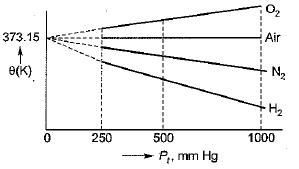QUESTION: 3

### As per international practice, the temperature interval from oxygen point to gold point is divided into three main parts. Which of the following temperature interval is not correct?

Solution:
QUESTION: 4

Match List-I with List-ll the following: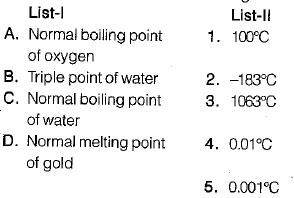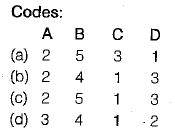Solution: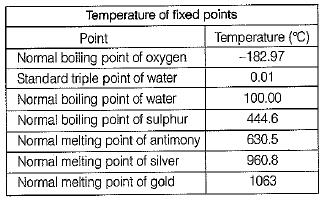QUESTION: 5

Which of the following is used for measuring high temperature beyond 1063°C?

Solution:

0 - 660°C → Platinum resistance thermocouple -190 to 0°C → Platinum-platinum/Rhodium Thermocouple > 1063°C → Planck’s law of thermal radiation.

QUESTION: 6

Match List-I (Type of thermometer) List-ll (Thermometric property) the following:

List-I
A. Mercury-in-glass
B. Thermocouple
C. Thermistor
D. Constant volume gas

List-ll
1. Pressure
2. Electrical resistance
3. Volume
4. Induced electric voltage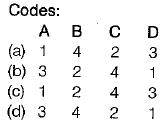Solution:
QUESTION: 7

Zeroth law of thermodynamics form the basis of measurement of

Solution:
• Zeroth law - concept of temperature
• First law - concept of internal energy
• Second law - concept of entropy
QUESTION: 8

The standard fixed point of thermometry is

Solution:
QUESTION: 9

Triple point temperature of water is

Solution:
QUESTION: 10

Experimental data obtained from a constant- volume-gas thermometer is shown is the figure below. The value of l in °C is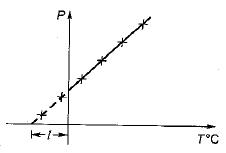Solution: